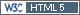## Do not trust in computers [Bioanalytics]

Dear DLabes!

» flame warin' a little bit with you.

Yeah, I like that!

» By the way, M\$-Excel is an extraordinary software in this way.

Definitely. If one has to use it for any reasons, he/she should follow the Ohlbe's suggestions.
BTW, even in M\$-Excel it's possible to get the correct answer (if `STDEV()` is avoided):
```    |       A       |       B     +---------------+-----------------------------   1 | 90000001      | =(A1-A\$4)^2   2 | 90000002      | =(A2-A\$4)^2   3 | 90000003      | =(A3-A\$4)^2   4 | =MEAN(A1:A3)  | =SUM(B1:B3)   5 | =STDEV(A1:A3) | =SQRT(1/(COUNT(A1:A3)-1)*B4)```
gives
```    |       A       |       B     +---------------+-----------------------------   1 | 90000001      | 1   2 | 90000002      | 0   3 | 90000003      | 1   4 | 90000002      | 2   5 | 0             | 1```

There are even better speadsheets around. OpenOffice Calc returns `1` if `STDEV()` is used, even with the oldest version I have on one of my machines, namely v2.0 from 2005.

Cheers,
Helmut SchützThe quality of responses received is directly proportional to the quality of the question asked. ☼
Science QuotesIng. Helmut Schütz